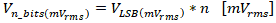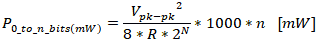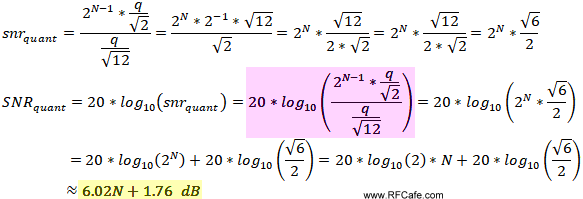# A/D Converter Calculations for RF ApplicationsVariable Definition VFS(pk) Full-scale peak input voltage VFS(pk-pk) Full-scale peak-to-peak input voltage VFS(rms) Full-scale input rms voltage PFS(mW) Full-scale input power in mW units at full-scale input voltage PFS(dBm) Full-scale input power in dBm units at full-scale input voltage fsample-rate Sampled analog input signal frequency in Hertz (Hz) VLSB(mVpk-pk) Peak-to-peak input voltage at one "q" (LSB) level; i.e., n=1 VLSB(mVprms) Rms input voltage at one "q" (LSB) level; i.e., n=1 Vn_bits(mVpk-pk) Peak-to-peak input voltage at n "q" levels; i.e., 0≤n≤2N Vn_bits(mVrms) Rms input voltage at n "q" levels; i.e., 0≤n≤2N ΔPn1_to-n2-_bits(dB) Difference in Pn1(dBm) and Pn2(dBm) expressed in units of dB snrquant Signal-to-noise ratio due to quantization (sampling) SNRquant snrquant expressed in decibels SNRaperture_jitter Signal-to-noise ratio due to aperture jitter NSDADC Noise spectral density expressed in decibels NFADC Noise figure expressed in decibels

These equations predict the RF electrical performance of an Analog-to-Digital Converter (ADC, A2D, A/D converter, etc.). Since A/D converters are often the last stage in a receiver chain, it is extremely useful to be able to predict the contribution for noise figure, signal-to-noise ratio, power levels, etc., since those values are needed for a complete cascade analysis. Lots of variations on the equations can be found across the Internet, so I have endeavored to reduce them to a few most common quantities. Calculations for dynamic range vary considerably amongst sources, so they are not presented here. It is best to consult device datasheets when possible for specific values.

Note: The following equations are valid for pure sinewave inputs with no DCoffset voltage. "R" is the input resistance in ohms. Be sure to note units and subscripts for both the input parameters and for the equations, or you will end up with really bad results.

Download RF Cafe Calculator Workbook to have the hard work done for you.

## Full Scale Voltage & Power

Power of a sinewave is calculated based on the root-mean-square (rms) value of the full-scale voltage. VFS(rms) calculated from the peak (pk) input voltage is:Using the peak-to-peak voltage (pk-pk):Full-scale input power in units of milliwatts (mW) based on full-scale peak-to-peak input voltage is:Full-scale input power in units of dBm is:## Quantization Levels of an Analog-to-Digital Converter (ADC)

The value of a 1-bit (LSB, aka "q" level) voltage step anywhere between 0 and N bits for an N-bit ADC is:The value of an n-bit voltage step anywhere between 0 and N bits for an N-bit ADC is:Because decibel units represent a logarithmic and not linear relationship between of number of ADC bits ("n") and power level, a simple multiplication of "n" or "n2 - n1" times some fixed power reference value does not work. Instead, you must calculate the value in watts (or mW, nW, etc.) for each number of bits using the voltage at each level, then conversion to dBm units can be made for an absolute value at each bit count:The difference in Pn1(dBm) and Pn2(dBm) is expressed in units of dB as follows:## Signal-to-Noise Ratio (SNR) of an Analog-to-Digital Converter (ADC)

Most sources give the ideal quantization-based signal-to-noise ratio (SNR) equation as 6.02*N + 1.76 dB (yellow highlight below). A little more research turns up the source of that equation (purple highlight below). Here, I show the steps between purple and yellow, using common rules of logarithms and rules of exponents.Another equation exists for calculating SNR based on aperture jitter that looks like the following. Note in the graph to the right that the SNR goes negative - which is invalid - when finput_signal*taperture_jitter > 1/2π.It might be best to use the worst case of either SNRclock_jitter or SNRquant for system budget planning. Datasheets often provide SNR information, which should be used instead of any generalized equations.

## Noise Figure of an Analog-to-Digital Converter (ADC)

Probably the most difficult equation to find for an ADC is for noise figure (NF), which is typically the last component in a cascade calculation of a receiver chain. My source for the equation is a Texas Instruments (TI) document authored by Mr. Tommy Neu (it also appeared in MWJ). You need the SNR value either from the ADC datasheet or from the above equation is required. Noise spectral density (NSD) is also needed, so its equation is provided as follows. NSD units are formally W/Hz or, equivalently, V/√Hz; however, the equations are provided without units because of the manner in which bandwidth is absorbed into them in these simple forms.,

where:Finally, the noise figure (NF) is calculated, where kTB is −174 dBm/Hz:ExampleAn example for the ADS4149 from the aforementioned TI paper (page 4) helps to clarify the application.

fsample_rate = 250 Msps

N = 16 bits

Vpk-pk = 2 V

SNRfull_scale = 71.9 dB

kTBT=290K,B=1_Hz = -174 dBm

R = 200 ΩRelated Pages on RF Cafe

Updated August 16, 2019About RF CafeCopyright: 1996 - 2024Webmaster:    Kirt Blattenberger,    BSEE - KB3UON RF Cafe began life in 1996 as "RF Tools" in an AOL screen name web space totaling 2 MB. Its primary purpose was to provide me with ready access to commonly needed formulas and reference material while performing my work as an RF system and circuit design engineer. The World Wide Web (Internet) was largely an unknown entity at the time and bandwidth was a scarce commodity. Dial-up modems blazed along at 14.4 kbps while typing up your telephone line, and a nice lady's voice announced "You've Got Mail" when a new message arrived... All trademarks, copyrights, patents, and other rights of ownership to images and text used on the RF Cafe website are hereby acknowledged. My Hobby Website:  AirplanesAndRockets.comPlease Support RF Cafe by purchasing my  ridiculously low−priced products, all of which I created. These Are Available for Free